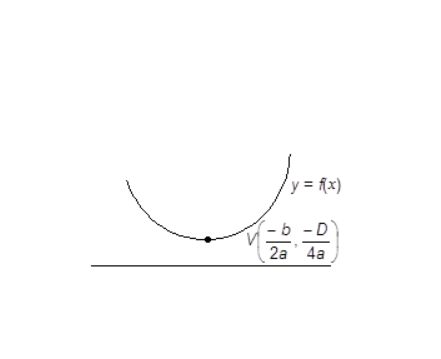# If the equationshave a common root, then a : b : c is : Option 1) 3 : 1 : 2 Option 2) 1 : 2 : 3 Option 3) 3 : 2 : 1 Option 4) 1 : 3 : 2

N neha
H Himanshu

As we have learned

Quadratic Expression Graph when a > 0 & D < 0 -

No Real and Equal root of

&

- whereinCondition for both roots common -

- wherein

&

are the 2 equations

For

Discriminant = 4-12 = -8 < 0

Both the roots are common as complex roots occur in conjugate

Option 1)

3 : 1 : 2

Option 2)

1 : 2 : 3

Option 3)

3 : 2 : 1

Option 4)

1 : 3 : 2

Exams
Articles
Questions